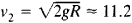# Elliptic Trajectory

The following article is from The Great Soviet Encyclopedia (1979). It might be outdated or ideologically biased.

## Elliptic Trajectory

one of the types of trajectories that may be followed by a mass point or the center of mass of a body moving in a Newtonian gravitational field. Suppose, for example a body located near the surface of the earth is given an initial velocity v0 < v2, wherekm/sec is the escape velocity (R is the earth’s radius, and g is the acceleration of gravity). If atmospheric resistance is disregarded, the body’s center of mass describes, in a first approximation, an elliptic trajectory.